Courses

# Test: Character Puzzles- 1

## 25 Questions MCQ Test Logical Reasoning (LR) and Data Interpretation (DI) | Test: Character Puzzles- 1

Description
This mock test of Test: Character Puzzles- 1 for LR helps you for every LR entrance exam. This contains 25 Multiple Choice Questions for LR Test: Character Puzzles- 1 (mcq) to study with solutions a complete question bank. The solved questions answers in this Test: Character Puzzles- 1 quiz give you a good mix of easy questions and tough questions. LR students definitely take this Test: Character Puzzles- 1 exercise for a better result in the exam. You can find other Test: Character Puzzles- 1 extra questions, long questions & short questions for LR on EduRev as well by searching above.
QUESTION: 1

### Which one will replace the question mark ?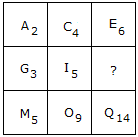Solution: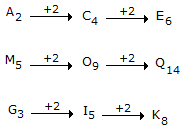How the number is obtained?

2 + 4 = 6

5 + 9 = 14

Similarly,

3 + 5 = 8

QUESTION: 2

### Which one will replace the question mark ?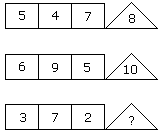Solution:

(5 + 4 + 7)/2 = 8

(6 + 9 + 5)/2 = 10

(3 + 7 + 2)/2 = 6.

QUESTION: 3

### Which one will replace the question mark ?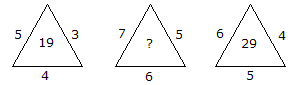Solution:

(5 x 3) + 4 = 19

and (6 x 4) + 5 = 29

Therefore, (7 x 5) + 6 = 41

QUESTION: 4

Which one will replace the question mark ?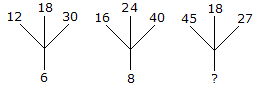Solution:

(12 + 18 + 30)/10 = 6

(16 + 24 + 40)/10 = 8

Similarly, (45 + 18 + 27)/10 = 9.

QUESTION: 5

Which one will replace the question mark ?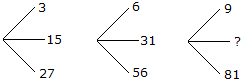Solution:

(15 x 2 - 3) = 27,

(31 x 2 - 6) = 56

and (45 x 2 - 9) = 81

QUESTION: 6

Which one will replace the question mark ?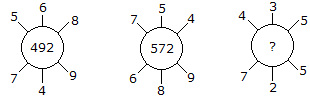Solution:

(5 x 6 x 8) + (7 x 4 x 9) = 492

and (7 x 5 x 4) + (6 x 8 x 9) = 572

Therefore (4 x 3 x 5) + (7 x 2 x 5) = 130.

QUESTION: 7

Which one will replace the question mark ?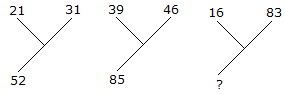Solution:

21 + 31 = 52

and 39 + 46 = 85

Therefore, 16 + 83 = 99.

QUESTION: 8

Which one will replace the question mark ?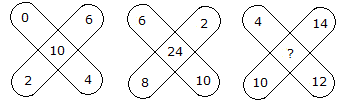Solution:

(0 + 2 + 6 + 4) - 2 = 10

and (6 + 2 + 10 + 8) - 2 = 24

Therefore, (4 + 14 + 12 + 10) - 2 = 38.

QUESTION: 9

Which one will replace the question mark ?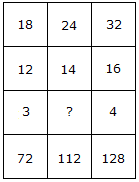Solution:

(18 x 12)/3 = 72

and (32 x 16)/4 = 128

Therefore, (24 x 14)/? = 112

(336/?) = 112

? = (336/112)

? = 3.

QUESTION: 10

Which one will replace the question mark ?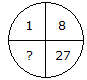Solution:

(1)3 = 1, (2)3 = 8, (3)3 = 27

Therefore, (4)3 = 64.

QUESTION: 11

Which one will replace the question mark ?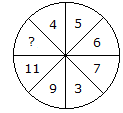Solution:

Sum of numbers in lower half of the circle = 11 + 9 + 3 + 7 = 30

Sum of numbers in upper half of the circle = ? + 4 + 5 + 6 = ? + 15

Upper half = Lower half

30 = ? + 15

? = 30 - 15

? = 15.

QUESTION: 12

Which one will replace the question mark ?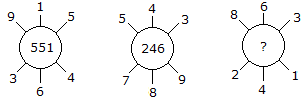Solution:

915 - 364 = 551

and 789 - 543 = 246

Similarly, 863 - 241 = 622.

QUESTION: 13

Which one will replace the question mark ?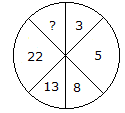Solution: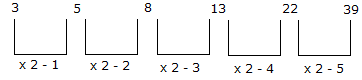QUESTION: 14

Which one will replace the question mark ?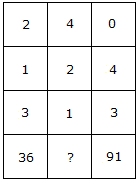Solution:

(2)3 + (1)3 + (3)3 = 36

and (0)3 + (4)3 + (3)3 = 91

Therefore, (4)3 + (2)3 + (1)3 = 73.

QUESTION: 15

Which one will replace the question mark ?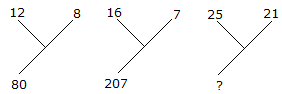Solution:

(12)2 - (8)2 = 80

and (16)2 - (7)2 = 207

Therefore (25)2 - (21)2 = 184.

QUESTION: 16

Which one will replace the question mark ?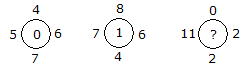Solution:

(6 + 5) - (7 + 4) = 0

and (7 + 6) - (8 + 4) = 1

Therefore (11 + 2) - (2 + 0) = 11.

QUESTION: 17

Which one will replace the question mark ?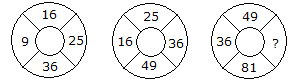Solution:

The numbers are squared in ascending order

In first circle,

(3)2 = 9

(4)2 = 16

(5)2 = 25

(6)2 = 36.

In second circle,

(4)2 = 16

(5)2 = 25

(6)2 = 36

(7)2 = 49.

In third circle,

(6)2 = 36

(7)2 = 49

(8)2 = 64

(9)2 = 81.

QUESTION: 18

Which one will replace the question mark ?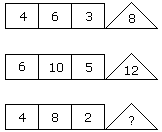Solution:

(4 x 6) % 3 = 8

(6 x 10) % 5 = 12

(4 x 8) % 2 = 16.

QUESTION: 19

Which one will replace the question mark ?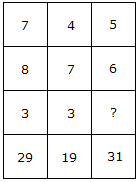Solution:

(7 x 3) + 8 = 29

(4 x 3) + 7 = 19

(5 x ?) + 6 = 31

? = 5.

QUESTION: 20

Which one will replace the question mark ?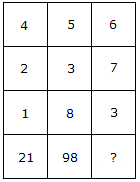Solution:

(4)2 + (2)2 + (1)2 = 21

and (5)2 + (3)2 + (8)2 = 98

Therefore (6)2 + (7)2 + (3)2 = 94.

QUESTION: 21

Which one will replace the question mark ?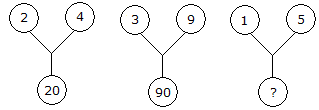Solution:

(2)2 + (4)2 = 20

and (3)2 + (9)2 = 90

Therefore (1)2 + (5)2 = 26.

QUESTION: 22

Which one will replace the question mark ?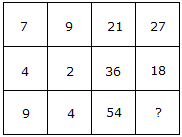Solution:

(7 x 3) = 21 and (9 x 3) = 27

and (4 x 9) = 36 and (2 x 9) = 18

Therefore (9 x 6) = 54 and (4 x 6) = 24.

QUESTION: 23

Which one will replace the question mark ?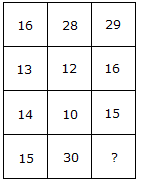Solution:

(16 + 13) = (14 + 15)

and (28 + 12) = (10 + 30)

Therefore (29 + 16) = (15 + 30).

QUESTION: 24

Which one will replace the question mark ?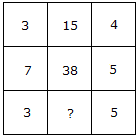Solution:

(3 x 4) + 3 = 15

and (7 x 5) + 3 = 38

Therefore (3 x 5) + 3 = 18.

QUESTION: 25

Which one will replace the question mark ?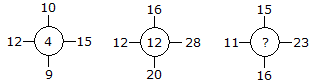Solution:

(15 - 12) + (10 - 9) = 4

(28 - 12) + (16 - 20) = 12

Similarly, (23 -11) + (15- 16) = 11.